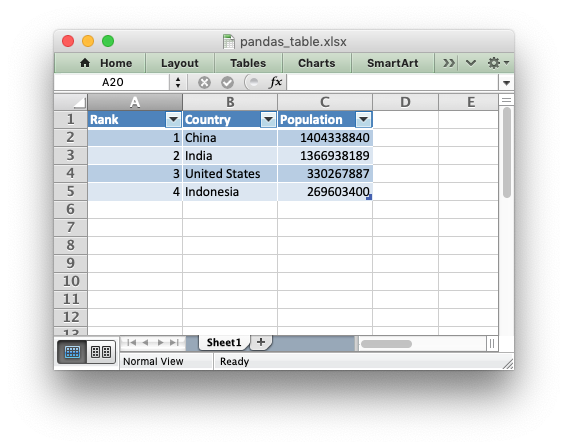# Example: Pandas Excel output with a worksheet table#

An example of inserting a Pandas dataframe into an Excel worksheet table file using Pandas and XlsxWriter.```##############################################################################
#
# An example of adding a dataframe to an worksheet table in an xlsx file
# using Pandas and XlsxWriter.
#
# Tables in Excel are used to group rows and columns of data into a single
# structure that can be referenced in a formula or formatted collectively.
#
# Copyright 2013-2023, John McNamara, jmcnamara@cpan.org
#

import pandas as pd

# Create a Pandas dataframe from some data.
df = pd.DataFrame(
{
"Country": ["China", "India", "United States", "Indonesia"],
"Population": [1404338840, 1366938189, 330267887, 269603400],
"Rank": [1, 2, 3, 4],
}
)

# Order the columns if necessary.
df = df[["Rank", "Country", "Population"]]

# Create a Pandas Excel writer using XlsxWriter as the engine.
writer = pd.ExcelWriter("pandas_table.xlsx", engine="xlsxwriter")

# Write the dataframe data to XlsxWriter. Turn off the default header and
# index and skip one row to allow us to insert a user defined header.

# Get the xlsxwriter workbook and worksheet objects.
workbook = writer.book
worksheet = writer.sheets["Sheet1"]

# Get the dimensions of the dataframe.
(max_row, max_col) = df.shape